# 1.6 Case Study: Random Web Surfer

Communicating across the web has become an integral part of everyday life. This communication is enabled in part by scientific studies of the structure of the web. We consider a simple model, known as the random surfer model. We consider the web to be a fixed set of pages, with each page containing a fixed set of hyperlinks, and each link a reference to some other page. We study what happens to a person (the random surfer) who randomly moves from page to page, either by typing a page name into the address bar or by clicking a link on the current page.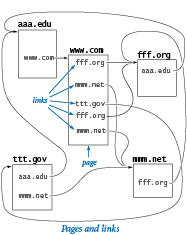## The Model

The first step in studying the random surfer model is to formulate it more precisely. The crux of the matter is to specify what it means to randomly move from page to page. The following intuitive 90-10 rule captures both methods of moving to a new page: Assume that 90 per cent of the time the random surfer clicks a random link on the current page (each link chosen with equal probability) and that 10 percent of the time the random surfer goes directly to a random page (all pages on the web chosen with equal probability).

You can immediately see that this model has flaws, because you know from your own experience that the behavior of a real web surfer is not quite so simple:

• No one chooses links or pages with equal probability.
• There is no real potential to surf directly to each page on the web.
• The 90-10 (or any fixed) breakdown is just a guess.
• It does not take the "back" button or bookmarks into account.
• We can only afford to work with a small sample of the web.

Despite these flaws, the model is sufficiently rich that computer scientists have learned a great deal about properties of the web by studying it. To appreciate the model, consider the small example above. Which page do you think the random surfer is most likely to visit?

## Input Format

We assume that there are n web pages, numbered from 0 to n-1, and we represent links with ordered pairs of such numbers, the first specifying the page containing the link and the second specifying the page to which it refers. Given these conventions, a straightforward input format for the random surfer problem is an input stream consisting of an integer (the value of n) followed by a sequence of pairs of integers (the representations of all the links).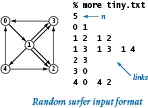The data files small.txt and medium.txt are two simple examples.

## Transition Matrix

We use a two-dimensional matrix, that we refer to as the transition matrix, to completely specify the behavior of the random surfer. With n web pages, we define an n-by-n matrix such that the entry in row i and column j is the probability that the random surfer moves to page j when on page i. The program transition.py is a filter that converts the list-of-links representation of a web model into a transition-matrix representation.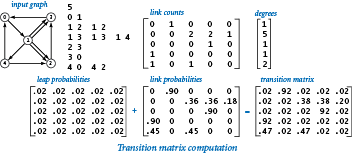## Simulation

The program randomsurfer.py simulates the behavior of the random surfer. It reads a transition matrix and surfs according to the rules, starting at page 0 and taking the number of moves as a command-line argument. It counts the number of times that the surfer visits each page. Dividing that count by the number of moves yields an estimate of the probability that a random surfer winds up on the page. This probability is known as the page's rank.

### One random move.

The key to the computation is the random move, which is specified by the transition matrix: each row represents a discrete probability distribution — the entries fully specify the behavior of the random surfer's next move, giving the probability of surfing to each page.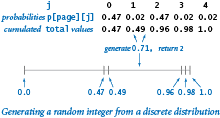### Markov chains.

The random process that describes the surfer's behavior is known as a Markov chain. Markov chains are widely applicable, well-studied, and have many remarkable and useful properties. For example, a basic limit theorem for Markov chains says that our surfer could start anywhere, because the probability that a random surfer eventually winds up on any particular page is the same for all starting pages! No matter where the surfer starts, the process eventually stabilizes to a point where further surfing provides no further information. This phenomenon is known as mixing.

### Page ranks.

The randomsurfer.py simulation is straightforward: it loops for the indicated number of moves, randomly surfing through the graph. Because of the mixing phenomenon, increasing the number of iterations gives increasingly accurate estimates of the probability that the surfer lands on each page (the page ranks). Accurate page rank estimates for the web are valuable in practice for many reasons. First, using them to put in order the pages that match the search criteria for web searches proved to be vastly more in line with people's expectations than previous methods.

### Visualizing the histogram.

With `stddraw`, it is also easy to create a visual representation that can give you a feeling for how the random surfer visit frequencies converge to the page ranks. randomsurferhistogram.py draws a frequency histogram that eventually stabilizes to the page ranks.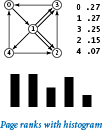## Mixing a Markov Chain

Directly simulating the behavior of a random surfer to understand the structure of the web is appealing, but can be too time consuming. Fortunately, we can compute the same quantity more efficiently by using linear algebra.

### Squaring a Markov chain.

What is the probability that the random surfer will move from page i to page j in two moves? The first move goes to an intermediate page k, so we calculate the probability of moving from i to k and then from k to j for all possible k and add up the results. This calculation is one that we have seen before — matrix-matrix multiplication.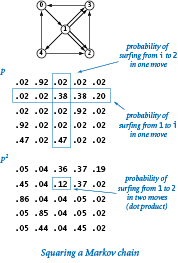### The power method.

We might then calculate the probabilities for three moves by multiplying by `p[][]` again, and for four moves by multiplying by `p[][]` yet again, and so forth. However, matrix-matrix multiplication is expensive, and we are actually interested in a vector-matrix calculation.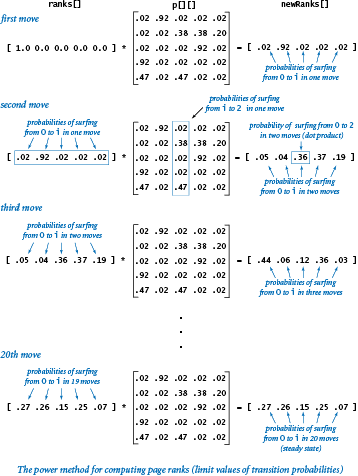The program markov.py is an implementation that you can use to check convergence for our example. For instance, it gets the same results (the page ranks accurate to two decimal places) as randomsurfer.py, but with just 20 vector-matrix multiplications.

## Lessons

Developing a full understanding of the random surfer model is beyond the scope of this booksite. Instead, our purpose is to show you an application that involves writing a bit more code than the short programs that we have been using to teach specific concepts. What specific lessons can we learn from this case study?

• We already have a full computational model. Primitive types of data and strings, conditionals and loops, arrays, and standard input/output enable you to address interesting problems of all sorts.
• Data-driven code is prevalent. The concept of using standard input and output streams and saving data in files is a powerful one.
• Accuracy can be elusive. It is a mistake to assume that a program produces accurate answers simply because it can write numbers to many decimal places of precision. Often, the most difficult challenge that we face is ensuring that we have accurate answers.
• Efficiency matters. It is also a mistake to assume that your computer is so fast that it can do any computation. The power method is a far more efficient way than direct simulation to to determine the page ranks, but it is still too slow for a huge matrix corresponding to all the pages on the web. That computation is enabled by better data structures and linear algebra.

#### Q & A

Q. What should row of transition matrix be if some page has no outlinks?

A. To make the matrix stochastic (all rows sum to 1), make that page equally likely to transition to every other page.

Q. How long until convergence of Markov?

A. Brin and Page report that only 50-100 iterations are need before the iterates converge. Convergence depends on the second largest eigenvalue of P λ2. The link structure of the Web is such λ2 is (approximately) equal to α = 0.9. Since .950 = .005153775207, we expect to have 2-3 digits of accuracy after 50 iterations.

Q. Any recommended readings on PageRank?

A. Here's a nice article from AMS describing PageRank.

Q. Why add the random page / teleportation component?

A. If not, random surfer can get stuck in part of the graph. More technical reason: makes the Markov chain ergodic.

#### Exercises

1. Modify transition.py to take the leap probability from the command line and use your modified version to examine the effect on page ranks of switching to an 80-20 rule or a 95-5 rule.

2. Modify transition.py to ignore the effect of multiple links. That is, if there are multiple links from one page to another, count them as one link. Create a small example that shows how this modification can change the order of page ranks.

3. Modify transition.py to handle pages with no outgoing links, by filling rows corresponding to such pages with the value 1/n.

4. The code fragment in randomsurfer.py that generates the random move fails if the probabilities in the row `p[page]` do not add up to 1. Explain what happens in that case, and suggest a way to fix the problem.

5. Determine, to within a factor of 10, the number of iterations required by randomsurfer.py to compute page ranks to four decimal places and to five decimal places for `small.txt`.

6. Determine the number of iterations required by markov.py to compute page ranks to three decimal places, to four decimal places, and to ten decimal places for `small.txt`.

7. Download the file medium.txt from the booksite (which reflects a 50-page example) and add to it links from page 23 to every other page. Observe the effect on the page ranks, and discuss the result.

8. Add to `medium.txt` (see the previous exercise) links to page 23 from every other page, observe the effect on the page ranks, and discuss the result.

9. Suppose that your page is page 23 in `medium.txt`. Is there a link that you could add from your page to some other page that would raise the rank of your page?

10. Suppose that your page is page 23 in `medium.txt`. Is there a link that you could add from your page to some other page that would lower the rank of that page?

11. Use transition.py and randomsurfer.py to determine the transition probabilities for the eight-page example shown below.

12. Use transition.py and markov.py to determine the transition probabilities for the eight-page example shown below.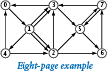#### Creative Exercises

1. Matrix squaring. Compose a program like markov.py that computes page ranks by repeatedly squaring the matrix, thus computing the sequence p, p2, p4, p8, p16, and so forth. Verify that all of the rows in the matrix converge to the same values.

2. Random web. Compose a generator for transition.py that takes as input a page count n and a link count m and writes to standard output n followed by m random pairs of integers from 0 to n-1.

3. Hubs and authorities. Add to your generator from the previous exercise a fixed number of hubs, which have links pointing to them from 10 percent of the pages, chosen at random, and authorities, which have links pointing from them to 10% of the pages. Compute page ranks. Which rank higher, hubs or authorities?

4. Page ranks. Design an array of pages and links where the highest-ranking page has fewer links pointing to it than some other page.

5. Hitting time. The hitting time for a page is the expected number of moves between times the random surfer visits the page. Run experiments to estimate page hitting times for `small.txt`, compare the results with page ranks, formulate a hypothesis about the relationship, and test your hypothesis on `medium.txt`. Fact: The hitting time = `1 / PageRank`.

6. Cover time. Compose a program that estimates the time required for the random surfer to visit every page at least once, starting from a random page.

7. Graphical simulation. Create a graphical simulation where the size of the dot representing each page is proportional to its rank. To make your program data-driven, design a file format that includes coordinates specifying where each page should be drawn. Test your program on `medium.txt`.